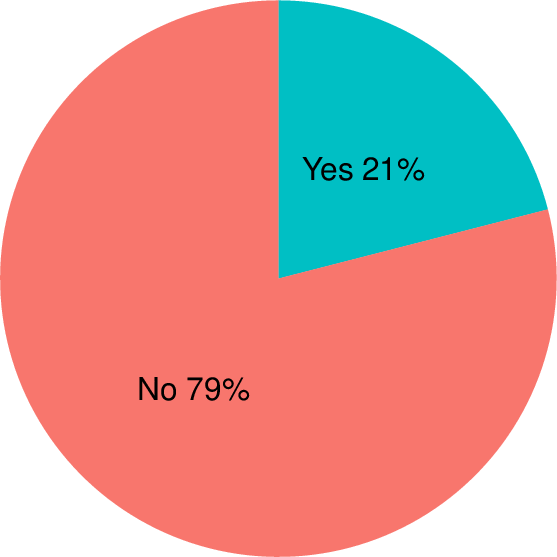Data Science Desktop Survival Guide by Graham WilliamsDesktop Survival Project Home Preface Data Science Introducing R R Constructs R Tasks R Strings R Read, Write, and Create Data Template Data Exploration Data Wrangling Data Visualisation Statistics ML Template ML Scenarios ML Activities ML Applications ML Algorithms Cluster Analysis Decision Trees Computer Vision Graph Data Privacy Literate Data Science Coding with Style Resources Bibliography Index

## Pie Chart

Rawds %>%   group_by(rain_tomorrow) %>%   count() %>%   ungroup()  %>%   mutate(per=round(`n`/sum(`n`), 2)) %>%   mutate(label=paste(rain_tomorrow, percent(per))) %>%   arrange(per) %>%   ggplot(aes(x=1, y=per, fill=rain_tomorrow)) +   geom_bar(stat="identity") +   coord_polar(theta='y') +   theme_void() +   theme(legend.position="none") +   geom_text(aes(x=1, y=cumsum(per)-per/2, label=label), size=8) A pie chart is a popular circular plot showing the relative proportions through angular slices. Generally, pie charts are not recommended, particularly for multiple wedges, because humans generally have difficulty perceiving the relative angular differences between slices. For two or three slices it may be argued that the pie chart is just fine, particularly if further information is provided, such as labelling the slices with their sizes. See also the illustrated tour and pie chart papers for explorations of the utility of pie charts.